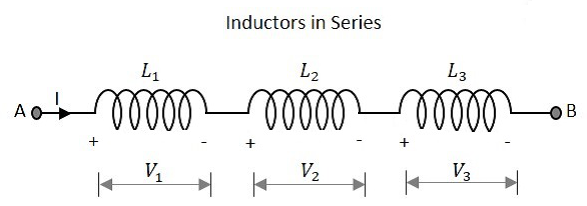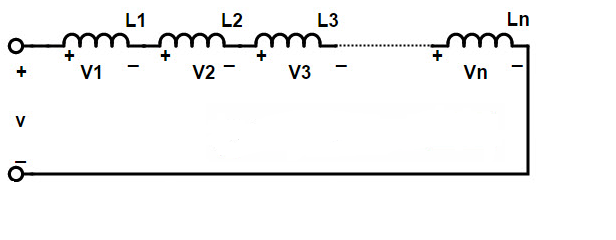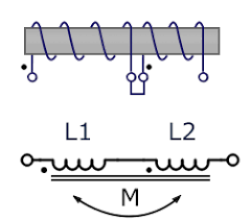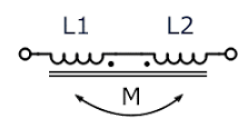# Inductors in Series Explained in Detail

Inductors are one of the passive elements ( others being resistors & capacitors) used in an electric circuit. It stores energy in the form of magnetic field.

Now inductors can broadly be connected in two ways

• Inductors in Series
• Inductors in Parallel

In this article, particularly we will be studying about the series connection of Inductors. We know that an inductor has a positive and a negative termial. So if the negative terminal of one is connected with the positive terminal of other then the connection is said to be made in series. Clearly, the current flowing through all inductors in series will be same.

### Inductors in Series Circuit

If the current flowing in all the connected inductors are same then the connection is series connection. The equivalent inductance of the inductors in series is equivalent to the sum of all the individual inductors in series.As shown above, the current is same in all three inductors,

i.e., I1 = I2 = I3

Also, we know that the voltage through an inductor is given by

V= L (di/dt)

### Equation of Equivalent Inductance

Applying Kirchoff’s law in the above circuit, we have

V = VL1 + VL2 + VL3

As, V = L (di/dt)

Therefore, V1 = L1 (dI1/dt)

V2 = L2 (dI2/dt)

V3 = L3 (dI3/dt)

putting the values of V1, V2 & V3 in the first equation, we get

V = L1 (dI1/dt) + L2 (dI2/dt) + L3 (dI3/dt)

Since current is same in all three, thus let I1 = I2 = I3 = I

V = L1 (dI/dt) + L2 (dI/dt) + L3 (dI/dt)

= (dI/dt) (L1 + L2 + L3)

= Lequi (dI/dt)

therefore, Lequi = (L1 + L2 + L3)

In general, Lequi = (L1 + L2 + L3 + ……..Ln)   [for ‘n’ inductors in series]‘n’ Inductors in Series

One point to remember here is that the equivalent inductance of two or more inductors connected in series is more than the largest inductance value among them.

### Example for Inductors in Series

Let four inductors are connected in series having values 10mH , 20mH, 30mH & 40mH, with no mutual inductance between them. Now to calculate the equivalent inductance of the combination is calculated by adding up all the individual inductances.

Therefore, Lequi = L1 + L2 + L3 + L4

Lequi = 10 + 20 + 30 + 40 = 100mH

Here also we can see that the largest value of individual inductance is 40mH and the equivalent is more that that (100mH).

### Mutually Connected Inductors in Series

When inductors are connected in series so that the magnetic field of one links with another, then the effect of mutual inductance either decreases or increases the total inductance depending on the magnetic coupling. The distance between the coils and their orientation to each other varies the effect of this mutual inductance.

Now, these mutually connected inductors can be broadly of two types:

• Opposing total inductance

If the current flowing through the coil and the magnetic flux produced are in the same direction then the coils are said to be cumulatively coupled. If the current direction is opposite to that of the magnetic flux produced then the coils are said to be connected in Differentially coupled.

### Cumulatively Coupled Series Inductors

As shown in fig below, when the current flowing between the two points through the cumulatively coupled coils has the same direction, therefore the voltage drops across each coil will be modified taking into consideration about the effect of mutual inducatance. The self inductances (L) will be the same here as well but there will be an addition of mutual inductance (M).Cumulatively Coupled Coils

Therefore, the total emf induced in these coils is given by

V = L1 (dI/dt) + L2 (dI/dt) 2M (dI/dt)

here, 2M represents the influence of L1 and L2 on each other.

As V = Lequi (di/dt)

putting it in above equation,

Lequi (di/dt) = L1 (dI/dt) + L2 (dI/dt) 2M (dI/dt)

dividing the above equation by dI/dt we get,

Lequi = L1 + L2 + 2M

If one of the coil is reversed, so as the same current flows through each coil but oin the opposite direction, the mutual inductance then the existing mutual inductance will have the cancelling effect on each coil as explained below.

### Differentially Coupled Series InductorsDifferentially Coupled Coils

The emf that is induced in coil one due to the effect of mutual inductance of coil two is in opposite direction when compared with the direction of self induced emf. This is because the same current now flows through each coil in opposite direction. Considering this cancelling effect, a minus sign is introduced with M when the magnetic field of two coils are differentially connected.

This gives us the final equation for calculating the total inductance of differentially connected coils as:

Lequi = L1 + L2 – 2M

Therefore, the final equation for inductively coupled inductors in series is given as:

Lequi = L1 + L2 ± 2M

### Example for Mutual Inductance Concept

Let two coils are connected in series having self inductances of 40mH and 50mH respectively. The total inductance of the combination was found to be 80mH. Find the value of mutual inductance that exists between the two coils between the two coils assuming that they are opposing each other.

we know that,

Lequi = L1 + L2 – 2M

substituting values for each variable, we have

80 = 40 + 50 -2(M)

2M = 90 – 80

therefore, M = 5mH

Let us take one more example to get more clear into the concept.

Suppose, two inductors of 30mH respectively are connected together in a series combination so that their magnetic fields aid each other giving cumulative coupling. Their mutual inductance is 5mH. Determine the total inductance of the series combination.

Lequi = L1 + L2 + 2M

= 30 + 30 + 2(5)

= 70mH

### Inductors in Series Summary

We know that the inductors can be connected in series to produce a total inductance value, Lequi  which is equal to the sum of the individuals inductors connected (same as in the case of resistors in series). However, when these are connected together then the value gets affected by the mutual inductance as well.

Mutual inductance case can be of two types, series aiding and series opposing. It depends on whether the coils are coupled cumulatively (in the same direction) or differentially (in the opposite direction). Accordingly the formula for the total inductance will change in both the cases.

AJAY DHEERAJ

Technical Content Developer

•
•
•
•
•
•